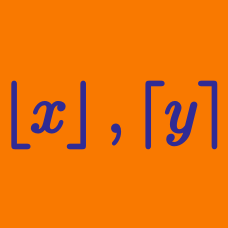Algebra

# Floor and Ceiling Functions: Level 3 Challenges

$\large {\lfloor -3.2 \rfloor =\, ? }$

Given that $x$ is a real number but not an integer, compute $\lfloor x \rfloor + \lfloor - x \rfloor$.

$\left\lfloor \left| \frac{2x-3}{4} \right| \right\rfloor = 5$

What is the largest negative integer $x$ that satisfies the equation above?

$\left\lfloor \frac { x }{ 1! } \right\rfloor +\left\lfloor \frac { x }{ 2! } \right\rfloor +\left\lfloor \frac { x }{ 3! } \right\rfloor =224$

Find the integer value of $x$ that satisfies the equation above.

Note: $\lfloor x \rfloor$ denotes the greatest integer that is smaller than or equal to $x$.

$\Large{\lceil x^2 \rceil=\lfloor 2|x| \rfloor}$ How many integers satisfy the above equation?

×